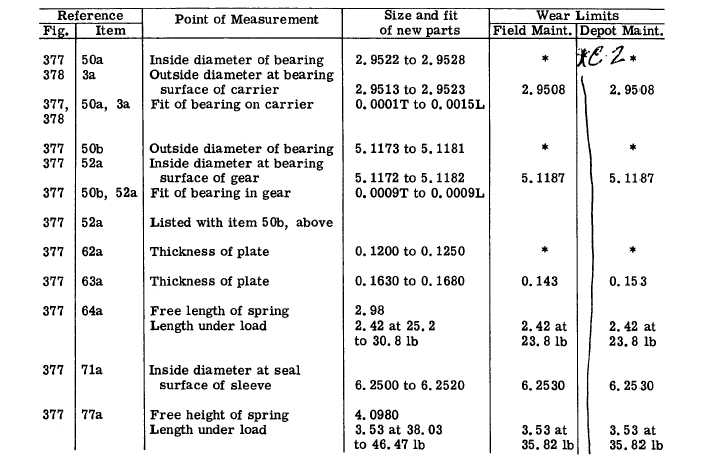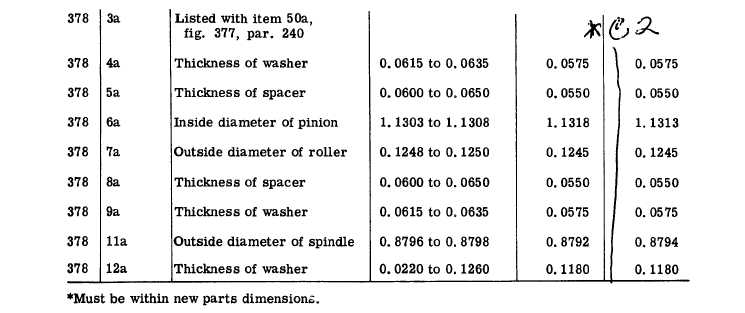L E F T - S T E E R   P L A N E T A R Y ,   A N D   B R A K E - R E P A I R   A N D   R E B U I L D   S T A N D A R D S
Custom Search*C2 *C2 C H A P   6 R E P A I R     A N D     R E B U I L D     S T A N D A R DS PAR  2 4 0 - 2 4 1 2 4 1 .    L E F T - S T E E R    P L A N E T A R Y ,    A N D    B R A K E - R E P A I R    A N D    R E B U I L D    S T A N D A R D S 2 1 4Integrated Publishing, Inc. - A (SDVOSB) Service Disabled Veteran Owned Small Business